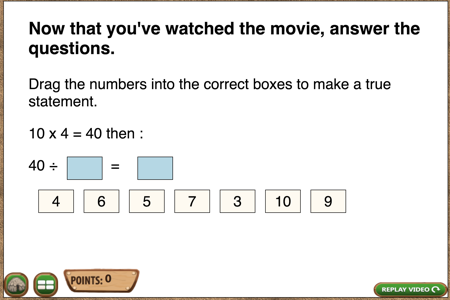# Why Good Education Isn’t Easy

Something known by all good teachers – but not all software developers – is that good education is complicated and difficult.

Let’s talk about a simple concept in math. Division is multiplication in reverse. If 4 x 7 = 28 then 28 /4 = 7.  Also, 28/7 = 4.

Easy, right?

### Recognition or recall versus application

To know whether a student understands this concept, I should give him or her a problem where it has to be APPLIED.  That is,  a student can memorize “division is multiplication in reverse”, spit that answer back at you and have no idea what it means.  All those teachers who studied Bloom’s taxonomy are nodding their heads. Many software developers are now googling Bloom’s taxonomy.

### Students Should Be Able to Apply a Concept to Multiple Problems

This is a simple programming task, you just need to remember to do it. A student can memorize or be told by a friend that the answer to problem 1 is 7 and 4, so you need to dynamically generate problems and not always give the same ones.

### Good Teachers Anticipate Wrong Answers

Let’s go back to this programming problem.If 10 x 4 = 40, application of the concept of division being multiplication in reverse would mean that the correct answer is 4 and 10. However, 5 x 8 also equals 40. This is not an application of the concept being taught and tested in this video, but it is a correct answer.

### Don’t Try to Teach Two Things at Once

With this problem, when students select 5 and 8, I could either mark it incorrect or correct, but, in either case, have a box float in with an explanation that while 40/8 = 5 , it doesn’t necessarily follow from 4 x 10 = 40.

You could argue that it does because 4 x2 x5 = 40  and 4 x2 = 8. You could argue that but I think you would be wrong, not about the mathematics of it but about trying to teach multiple concepts at the same time.

A second reason I think this would be wrong is that math is a pyramid. Students are generally learning about the distributive property after they have mastered the basics of multiplication.

To see how I coded the solution , check out my next post.